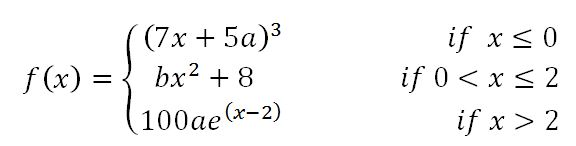# Continuity

### Continuity

The idea of continuity lies in many things we experience in our daily lives, for instance, the time it takes you to log into StudyPug and read this section. Limits and continuity are so related that we cannot only learn about one and ignore the other. We will learn about the relationship between these two concepts in this section.

#### Lessons

• Definition of "continuity" in everyday language
A function is continuous if it has no holes, asymptotes, or breaks. A continuous graph can be drawn without removing your pen from the paper.

• Definition of "continuity" in Calculus
A function $f$ is continuous at a number a, if: $\lim_{x \to a^-} f(x) = \lim_{x \to a^+} f(x) = f(a)$

• Polynomials are always continuous everywhere. Rational functions are continuous wherever the functions are defined; in other words, avoiding holes and asymptotes, rational functions are continuous everywhere. A function f is continuous at a number a, if and only if:
$\lim_{x \to a^-} f(x) = \lim_{x \to a^+} f(x) = f(a)$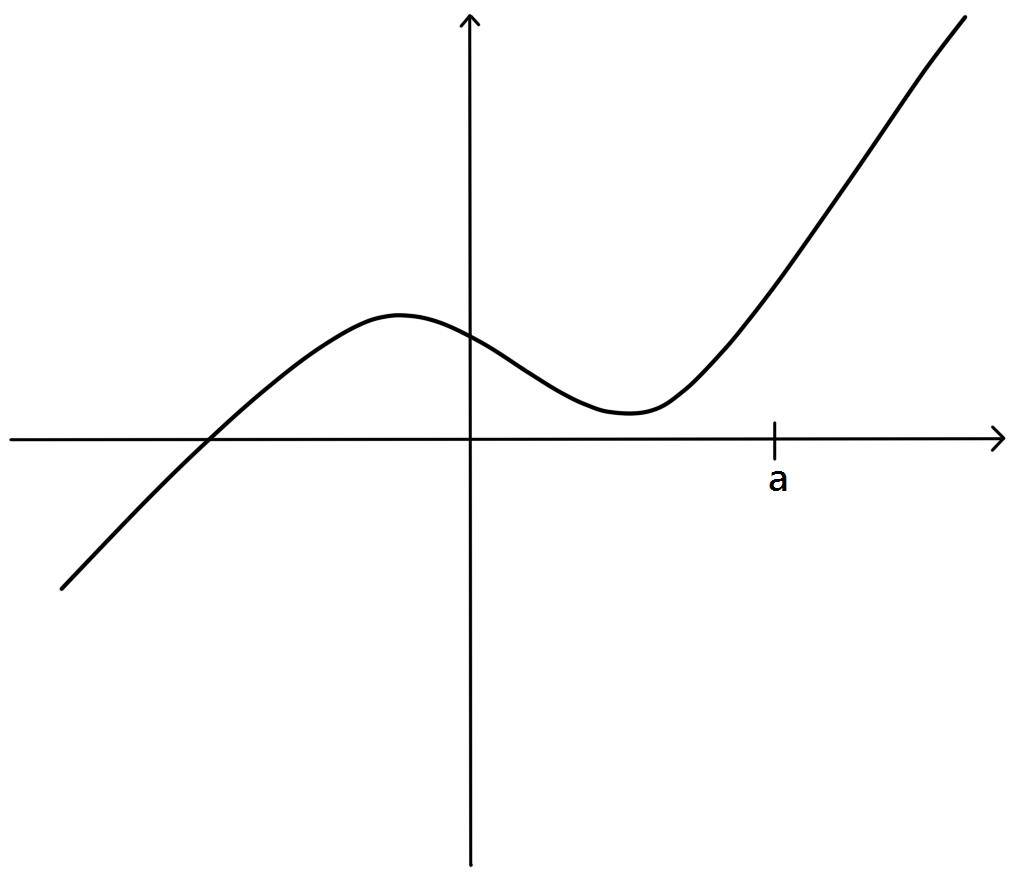In simple words, the graph of a continuous function has no break in it and can be drawn without lifting your pen from the paper.
• Introduction
Introduction to Continuity
a)
Discuss CONTINUITY in everyday language: a function whose graph has no break in it.

b)
Discuss CONTINUITY in the context of Calculus:
A function $f$ is continuous at a number a, if: $\lim_{x \to a^-} f(x) = \lim_{x \to a^+} f(x) = f(a)$

Classify different types of discontinuities: removable VS. infinite VS. jump

Analyze: rational function with a hole: $f(x)=\frac{(x-1)(x-2)}{(x-2)}$
VS.
rational function with an asymptote: $g(x)=\frac{1}{x+1}$
VS.
piecewise function: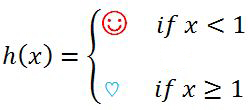c)
Lesson Overview - Continuity

• 1.
Discussing "Continuity" Graphically
The graph of a function $f$ is shown below.
a)
State the numbers at which $f$ is discontinuous.

b)
Explain and classify each discontinuity.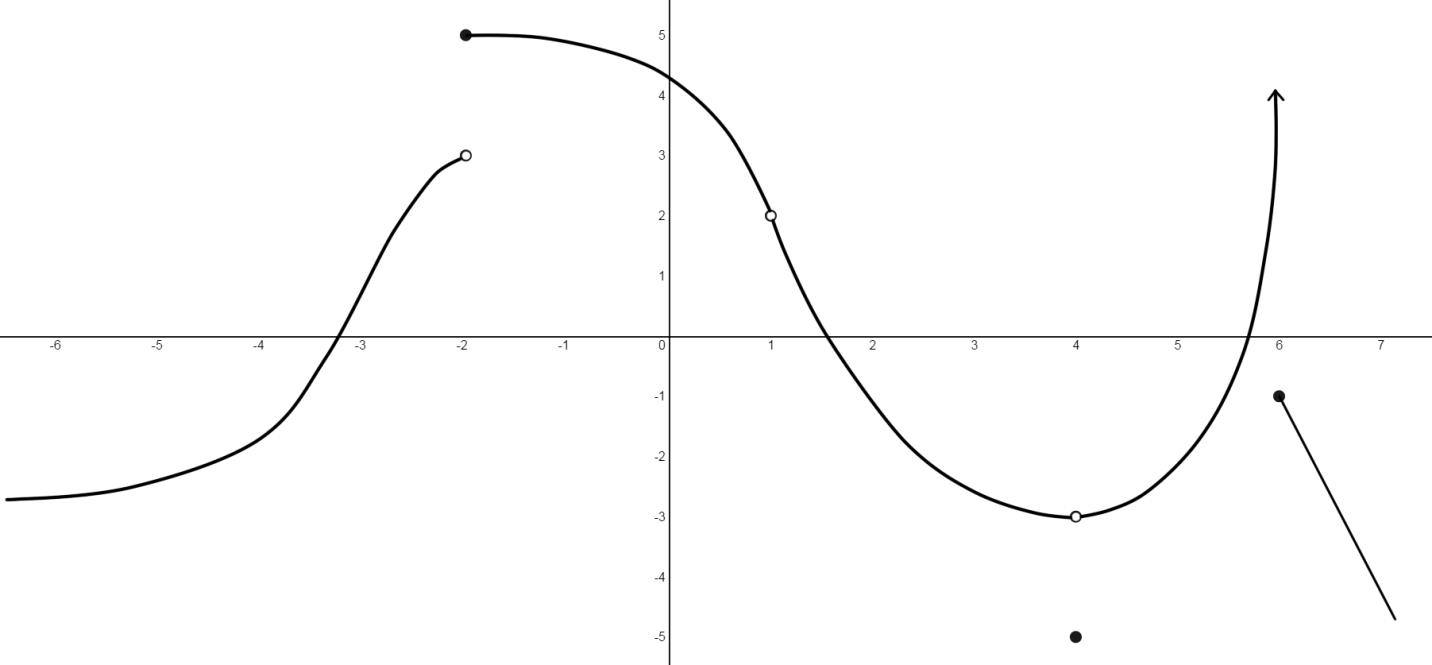• 2.
Detecting Discontinuities Are the following functions continuous at $x=3$?
i) $f(x)=\frac{x^2-2x-3}{x-3}$

ii)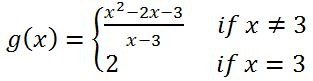iii)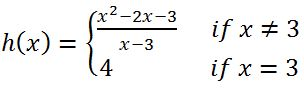• 3.
Discontinuities of Rational Functions (denominator=0)
Locate and classify each discontinuity of the function: $f(x)=\frac{x^2-9}{x^2+x-6}$

• 4.
Discussing "Continuity" Algebraically
Find the values of a and b that make the function $f$ continuous on ($-\infty$, $\infty$).